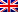# Trees and trees

Contenus

## Trees and arboresences

Trees and trees are special graphs often used to represent decision support, data, or for calculating complexity.

A tree is an organized set of nodes in which each node has one father and one, except a node which we call root. If a node p is the father of the knot f, so f is a son of p; if f has no son, so she is a sheet. It is possible to store any type of information in nodes or links.

## Terminology

A node is defined by its label and his sub-trees. It is therefore possible to represent a tree by a tuple <e,a1,…,Tok> in which e is the node label and Toi are its subtrees.

For example, calculators organize mathematical expressions according to operator precedence and depth: (y / 2 - x) * (75 + z) will be represented by <*, <- ,,>,>, <+ ,, >>. The operation is then done by a particular path of the tree:

• the descendants of a node are the nodes of its subtrees;
• an ancestor of a node is either its father or an ancestor of its father;
• the path from a node to the root is made up of all its ancestors;
• the brother of a node is a son of his father other than himself.

A tree is often represented by a graph to facilitate reading:

The nodes of a tree are distributed by depths (or levels). The depth 0 contains only the root, the depth 1 its children etc. The height of a tree is the number of depths, or the size of the longest path from a node to the root.

## Definition: graph theory

Given an undirected graph comprising not vertices, the following properties are equivalent:

1. The graph is connected and without cycle,
2. The graph is cycleless and has n-1 edges,
3. The graph is connected and admits n-1 edges,
4. The graph is cycleless, and by adding an edge, then we create one and only one elementary cycle,
5. The graph is connected, and by removing any edge it is no longer connected,
6. There is one chain and only one between all pairs of vertices.

A tree is a directed graph without circuit admitting a root such that for any other vertex there is a unique path from the root to this vertex. A tree has properties similar to a tree.

## Binary tree

In a binary tree, each node has a left child and a right child, which can be zero subtrees. A binary tree is complete if all of its leaves have the same depth and all of its nodes that are not leaves have two children.

Let us determine the total number of leaves and nodes of a complete binary tree.

At depth 0 there is a leaf, the root. Suppose the complete binary tree has 2(h-1) leaves up to the task h. So, at the height h + 1, each of these leaves becomes a node with two threads, so we have a number of leaves of 2 * 2(h-1) = 2h. CQFD.

In addition, the number of nodes of the complete binary graph is equal to the sum of the leaf number of the complete binary trees of lower height. We deduce that the total number of node is ∑(i = 0)(h-1) 2i = 2h -1. Conversely, if a complete binary graph has n nodes, then its height is according to the preceding formula log2 (n) +1. We deduce that any binary tree has at least log height2 (n) +1.

A balanced binary tree or AVL tree is a binary tree such that the heights of the two subtrees of any node in the tree differ by at most 1. A subtree of an AVL tree is also an AVL tree.

The indicator on the vertices shows the difference between the height of the left subtree and the height of the right subtree.

When the tree is unbalanced, it is then necessary to swap the parent vertices and the root while maintaining the order of the sub-trees (see the continuation on research trees).

We can enlarge the definition on trees of higher degree (ternary tree etc). Only the coefficient 2 is modified according to the number of wires defined by the type of shaft.

## Research tree

A search tree is a data structure making it possible to represent a set of values if we have an order relation on them. The standard operations on search trees are: inserting, deleting or finding a value. These operations are inexpensive if the shaft is balanced. In order to facilitate understanding, we will work on binary search trees (ABR).

Let be a set of values E provided with an order relation, and either TO a binary tree. The tree TO is an ABR of E if for any node p of TO, the value of p is strictly greater than the values of its left subtree, and is strictly smaller than the values in its right subtree; provided the values are unique. The values are called keys.

The smallest value is the last left descendant of the root, and the largest is the last right descendant of the root. Other logical criteria can be deduced from the definition:

The three actions are then carried out through ABR routes.FRFRENRUES